# 7. [MT, p. 210] Investigate whether or not the system u(x, y, z) = x +...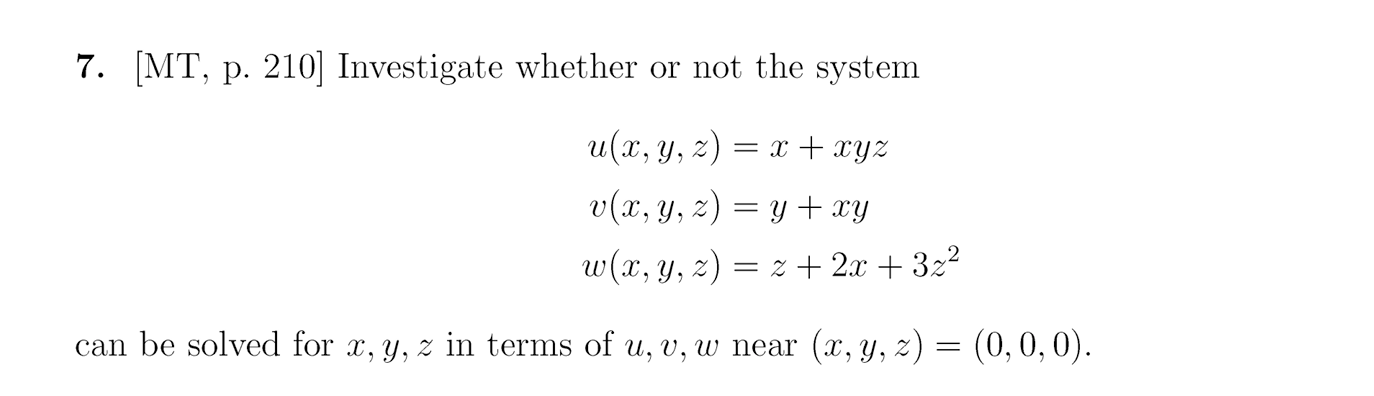7. [MT, p. 210] Investigate whether or not the system u(x, y, z) = x + xyz V(x, y, z) y + xy W(x, y, z) = 2 + 2x + 322 = can be solved for x, y, z in terms of u, v, w near (x, y, z) = (0,0,0).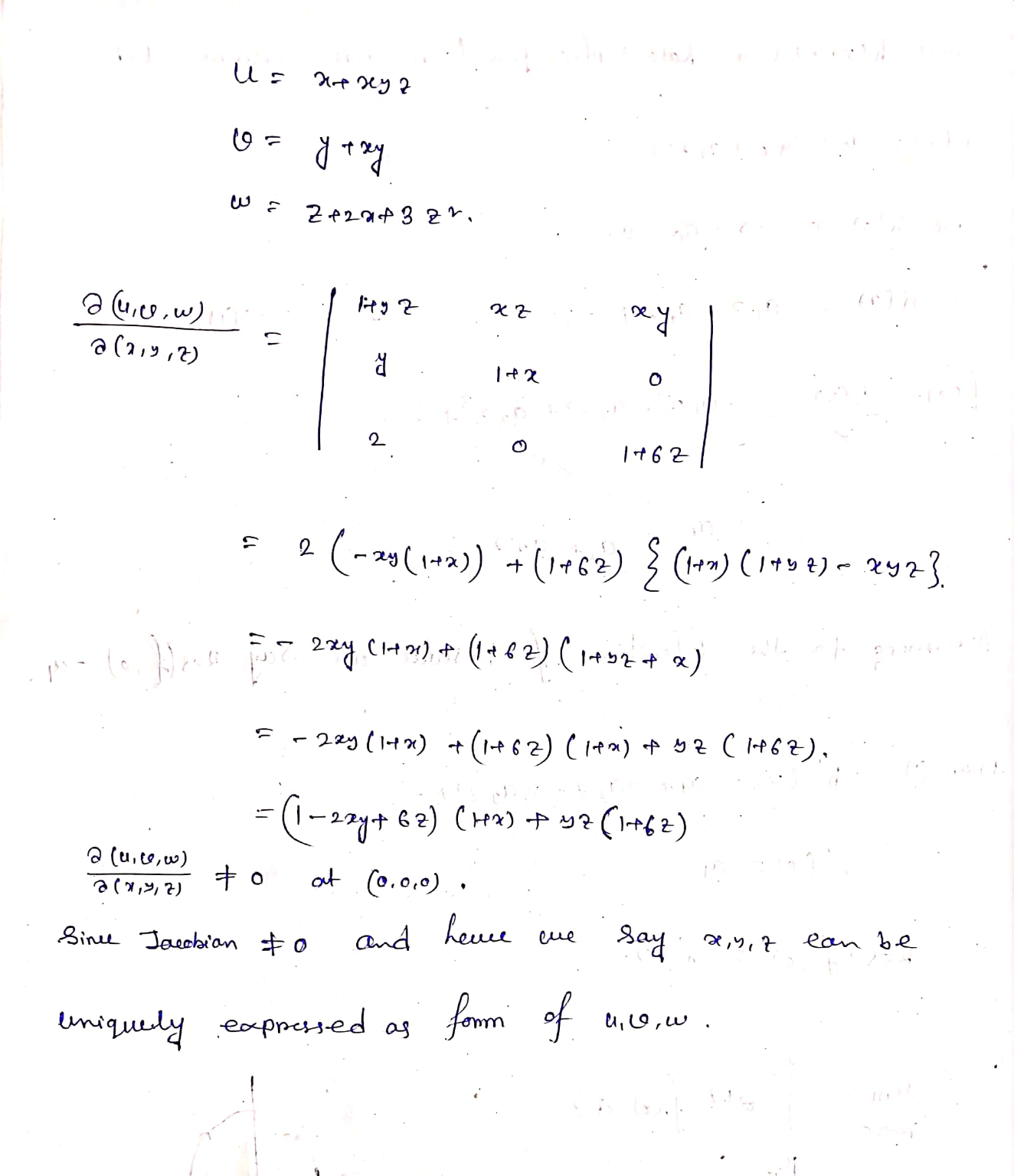##### Add Answer of: 7. [MT, p. 210] Investigate whether or not the system u(x, y, z) = x +...
Similar Homework Help Questions
• ### The following system can be solved? for u, u and w in ter ms of 1.J and near χ = y = z = 0. u = u = 0 and The following system can be solved? for u, u and w in ter ms of 1.J and near χ = y = z =...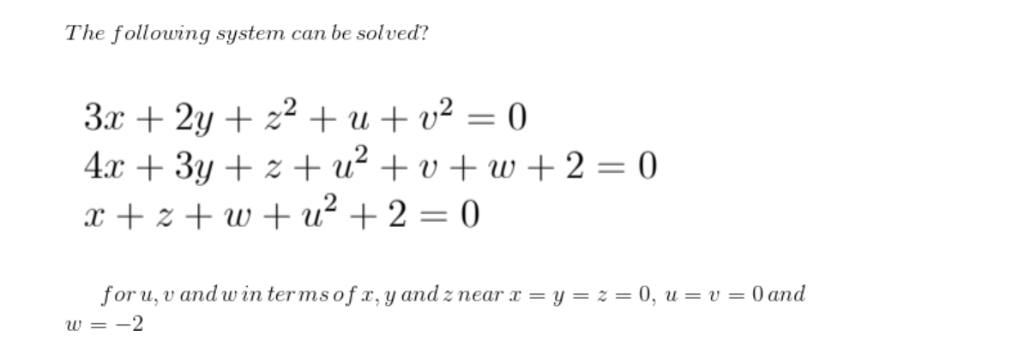The following system can be solved? for u, u and w in ter ms of 1.J and near χ = y = z = 0. u = u = 0 and The following system can be solved? for u, u and w in ter ms of 1.J and near χ = y = z = 0. u = u = 0 and

• ### please help with these questions 7. Find fryzx, for f(x, y, z) = 3 + 2?x...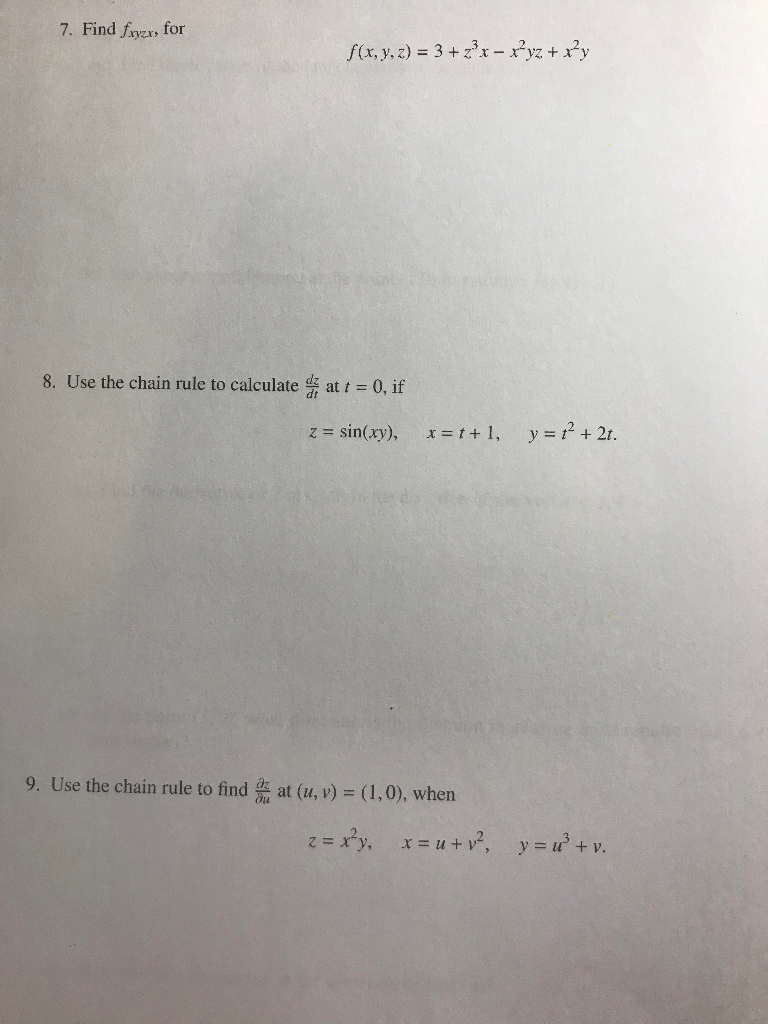please help with these questions 7. Find fryzx, for f(x, y, z) = 3 + 2?x – xyz + x+y 8. Use the chain rule to calculate that t = 0, if z = sin(xy), x = 1+1, y = 12 + 2t. 9. Use the chain rule to find us at (u, v) = (1,0), when z = xy, x = u +v?, y = x + v.

• ### 1. Suppose that E(X) E(Y) E(Z) 2 Y and Z are independent, Cov(X, Y) V(X) V(Z)...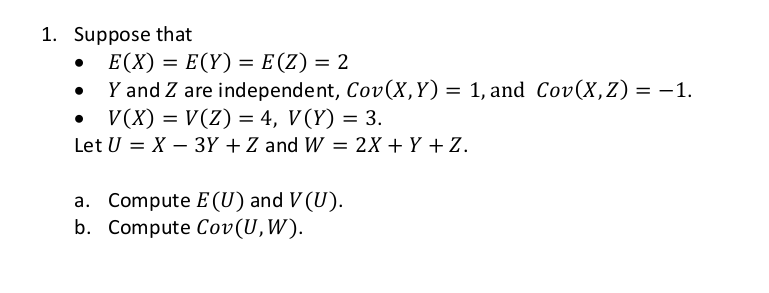1. Suppose that E(X) E(Y) E(Z) 2 Y and Z are independent, Cov(X, Y) V(X) V(Z) 4, V(Y) = 3 Let U X 3Y +Z and W = 2X + Y + Z 1, and Cov(X, Z) = -1 Compute E(U) and V (U) b. Compute Cov(U, W). а.

• ### Calculus, implicit function theorem

The following system of three nonlinear algebraic equations is to be solved forx, y, z as functions of the variables u, v,w:u = x + y + zv = x2 + y2 + z2w = x3 + y3 + z3a. Prove or find a counter example: for each (u,v,w) near (0,2,0), there is a uniquesolution (x,y,z) near (-1,0, 1).b. Is the Implicit Function Theorem applicable for (u,v,w) near (2, 4, 8) and(x,y, z) near (0, 0, 2)?

• ### For U = {q, r, s, t, u, v, w, x, y, z} A = {q, s, u, w, y} B = {q, s, y, z} C = {v, w, x, y, z} identify the following

For U = {q, r, s, t, u, v, w, x, y, z}A = {q, s, u, w, y}B = {q, s, y, z}C = {v, w, x, y, z}identify the following.5) CA) {v, w, x, y, z}B) {q, r, s, t, u}C) { }D) {v, z}

• ### Define an equivalence relation on R by (x,y,z) ∼ (u,v,w) whenever x +y +z = u...

Define an equivalence relation on R by (x,y,z) ∼ (u,v,w) whenever x +y +z = u +v +w . Describe the equivalence classes.

• ### solved item S, T, U, V, W, X, Y, Z The demand for subassembly S is...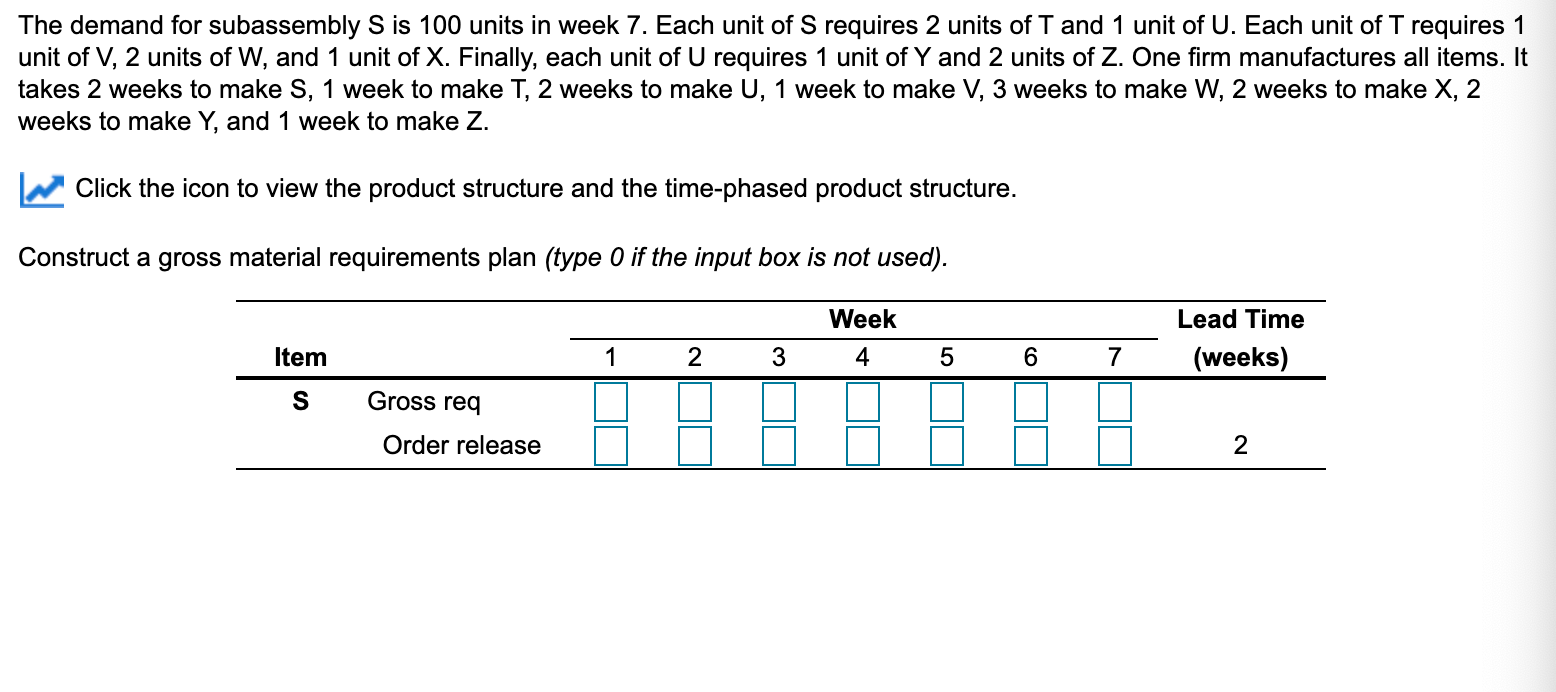solved item S, T, U, V, W, X, Y, Z The demand for subassembly S is 100 units in week 7. Each unit of S requires 2 units of T and 1 unit of U. Each unit of T requires 1 unit of V, 2 units of W, and 1 unit of X. Finally, each unit of U requires 1 unit of Y and 2 units of Z. One firm manufactures all items. It takes 2 weeks to make S,...

• ### can you please answer all of them please need it for a review F(x y, z)...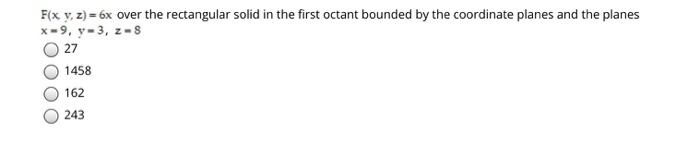can you please answer all of them please need it for a review F(x y, z) = 6x over the rectangular solid in the first octant bounded by the coordinate planes and the planes X-9, y-3, 2-S 27 1458 162 243 Find the center of mass of a thin triangular plate bounded by the coordinate axes and the line x + y = 4 if o(x, y) = x + y. 5 5 -3.73 . Oz Find the center of...

• ### let U= {q,r,s,t,u,v,w,x,y,z}; A= {q,s,u,w,y};and C={v,w,x,y,z,}; list the members of the indicated set , using set braces A'u B A

4.let U= {q,r,s,t,u,v,w,x,y,z}; A= {q,s,u,w,y};and C={v,w,x,y,z,}; list the members of the indicated set , using set braces A'u B A.{Q,R,S,T,V,X,Y,Z} B.{S,U,W} C.{R,S,T,U,V,W,X,Z} D.{Q,S,T,U,V,W,X,Y}

• ### 7. Assume X gammala, y) and Y-gamma(8,7) are independent. (a) Show that U = X +...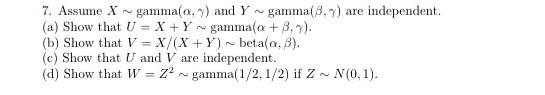7. Assume X gammala, y) and Y-gamma(8,7) are independent. (a) Show that U = X + Y gamma(a +.). (b) Show that V = X/(X+Y) beta(a. 8). (c) Show that U and V are independent. (d) Show that W = 72 gamma(1/2, 1/2) if Z N (0,1).

Free Homework App